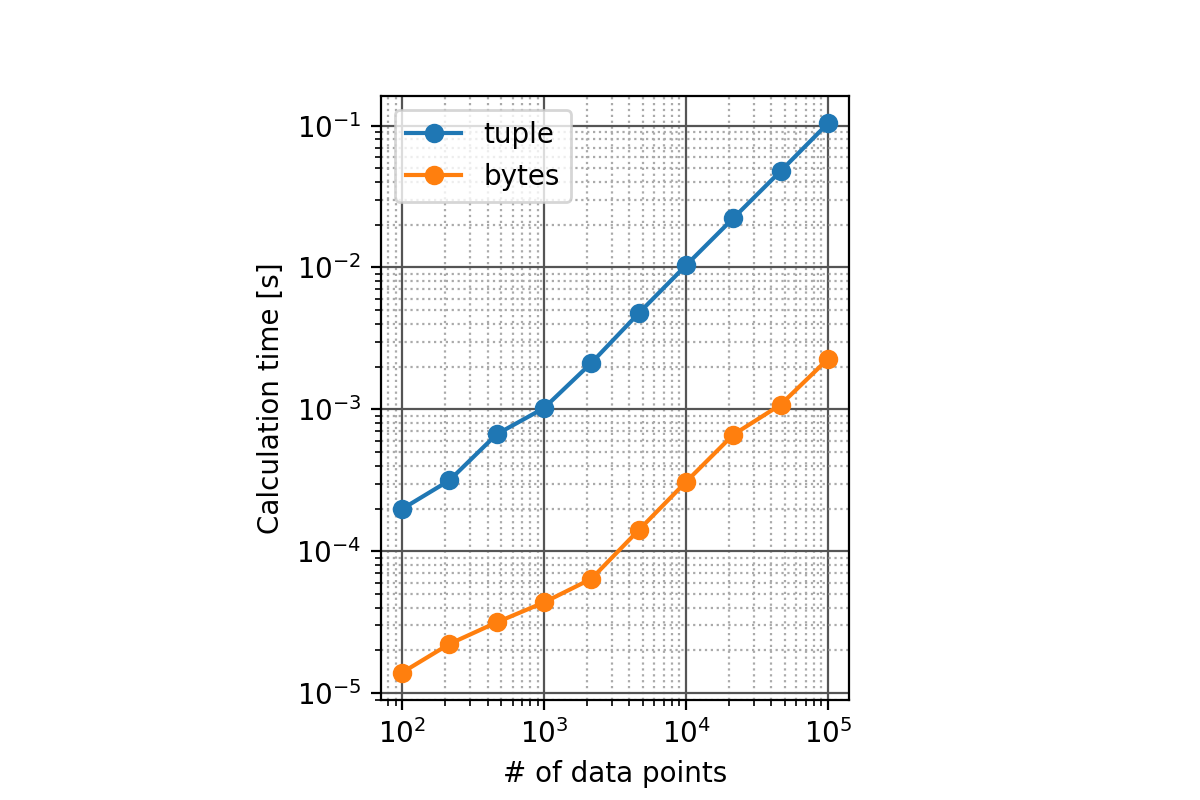# What is this

A way to convert a regular ndarray defined with a single type to a structured array.

### Motivation

--Multiple data is time series (2D array) and I want to access it like a dictionary using key --I want to complete it with just numpy --There was an article that you can use record array (Stack Overflow : Converting a 2D numpy array to a structured array), but recarray still remains because of backward compatibility with numpy, but structured array There seems to be a discussion that is newer (Stack Overflow : NumPy “record array” or “structured array” or “recarray”), so I will implement it with a structured array.

# Method 1: Via a list of tuples

--The numpy docs tell you to pass the data in a list of tuples - Numpy : Structured array : Assignment from Python Native Types (Tuples)

### Code example

--For example, the code will be as follows

``````import numpy

# d1, d2,Suppose you have 3 data in d3
d1 = numpy.arange(0, 1000, dtype='int32')
d2 = numpy.arange(1000, 2000, dtype='int32')
d3 = numpy.arange(2000, 3000, dtype='int32')

#Stick together
d = numpy.array([d1, d2, d3]).T

#d looks like this
# array([[   0, 1000, 2000],
#        [   1, 1001, 2001],
#        [   2, 1002, 2002],
#        ...,
#        [ 997, 1997, 2997],
#        [ 998, 1998, 2998],
#        [ 999, 1999, 2999]], dtype=int32)

#When defining dtype
dtype1 = [
('d1', 'int32'),
('d2', 'int32'),
('d3', 'int32'),
]

#Convert to structured array
sa1 = numpy.array(list(map(tuple, d)), dtype=dtype1)

#sa1 looks like this
# array([(  0, 1000, 2000), (  1, 1001, 2001), (  2, 1002, 2002),
#        (  3, 1003, 2003), (  4, 1004, 2004), (  5, 1005, 2005),
#        (  6, 1006, 2006), (  7, 1007, 2007), (  8, 1008, 2008),
#        ...
#        (993, 1993, 2993), (994, 1994, 2994), (995, 1995, 2995),
#        (996, 1996, 2996), (997, 1997, 2997), (998, 1998, 2998),
#        (999, 1999, 2999)],
#        dtype=[('d1', '<i4'), ('d2', '<i4'), ('d3', '<i4')])

#You can now access individual data with a key
sa1['d1']
# array([  0,   1,   2,   3,   4,   5,   6,   7,   8,   9,  10,  11,  12,
#         13,  14,  15,  16,  17,  18,  19,  20,  21,  22,  23,  24,  25,
#         ...
#        975, 976, 977, 978, 979, 980, 981, 982, 983, 984, 985, 986, 987,
#        988, 989, 990, 991, 992, 993, 994, 995, 996, 997, 998, 999],
#       dtype=int32)
``````

### performance

--I'm converting from numpy.ndarray to tuple and it's a bit slow ――It took about 3 ms in my environment

``````%time numpy.array(list(map(tuple, d)), dtype=dtype1)
# CPU times: user 2.63 ms, sys: 0 ns, total: 2.63 ms
# Wall time: 2.64 ms
``````

# Method 2: Go through the buffer

――I want to make it faster --Structured array is very convenient to open a binary file as it is by using numpy.frombuffer (). -Which is faster to process binary data --Kitsune Gadget - Stack Overflow : Reading in numpy array from buffer with different data types without copying array --If you take out the data in the memory of ndarray with tobytes () and reinterpret it with frombuffer (), it must be fast.

### Code example

--For example, the code will be as follows

``````import numpy

#make data
d1 = numpy.arange(0, 1000, dtype='int32')
d2 = numpy.arange(1000, 2000, dtype='int32')
d3 = numpy.arange(2000, 3000, dtype='int32')

d = numpy.array([d1, d2, d3]).T

#Define dtype
dtype1 = [
('d1', 'int32'),
('d2', 'int32'),
('d3', 'int32'),
]

###So far, it's the same as method 1.###

#Convert to structured array
sa2 = numpy.frombuffer(d.tobytes(), dtype=dtype1)

#The values ​​of sa1 and sa2 are exactly the same
all(sa2 == sa1)
# >> True
``````

### performance

――It became 80 us in the environment at hand ――It is about 30 times faster than via tuple. ――I am very satisfied

``````%time numpy.frombuffer(d.tobytes(), dtype=dtype1)
# CPU times: user 75 µs, sys: 0 ns, total: 75 µs
# Wall time: 83.9 µs
``````

# Compare a little more seriously

--I tried to measure the calculation time of method 1 and method 2. --Change the number of data points n ――For each score, we calculated 100 times and averaged the required time.### code

``````import numpy
import time

dtype1 = [
('d1', 'int32'),
('d2', 'int32'),
('d3', 'int32'),
]

def run(num, func):
d = numpy.arange(num*3, dtype='int32').reshape((3, num)).T
t0 = time.time()
[func(d) for i in range(100)]
t1 = time.time()
return (t1 - t0) / 100

func1 = lambda x: numpy.array(list(map(tuple, x)), dtype=dtype1)
func2 = lambda x: numpy.frombuffer(x.tobytes(), dtype=dtype1)

#To measure
nums = numpy.logspace(2, 5, 10, dtype=int)
t1 = [run(i, func1) for i in nums]
t2 = [run(i, func2) for i in nums]

#Plot
import matplotlib.pyplot

fig = matplotlib.pyplot.figure()
ax = fig.add_subplot(111, aspect=1)
ax.plot(nums, t1, 'o-', label='tuple')
ax.plot(nums, t2, 'o-', label='bytes')
ax.set_xscale('log')
ax.set_yscale('log')
ax.set_xlabel('# of data points')
ax.set_ylabel('Calculation time [s]')
ax.grid(True, color='#555555')
ax.grid(True, which='minor', linestyle=':', color='#aaaaaa')
ax.legend()
fig.savefig('results.png', dpi=200)
``````